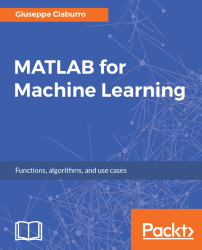•#### MATLAB for Machine Learning#### Overview of this book

MATLAB is the language of choice for many researchers and mathematics experts for machine learning. This book will help you build a foundation in machine learning using MATLAB for beginners. You’ll start by getting your system ready with t he MATLAB environment for machine learning and you’ll see how to easily interact with the Matlab workspace. We’ll then move on to data cleansing, mining and analyzing various data types in machine learning and you’ll see how to display data values on a plot. Next, you’ll get to know about the different types of regression techniques and how to apply them to your data using the MATLAB functions. You’ll understand the basic concepts of neural networks and perform data fitting, pattern recognition, and clustering analysis. Finally, you’ll explore feature selection and extraction techniques for dimensionality reduction for performance improvement. At the end of the book, you will learn to put it all together into real-world cases covering major machine learning algorithms and be comfortable in performing machine learning with MATLAB.
Title PageCreditsForewordwww.PacktPub.comCustomer FeedbackPrefaceFree Chapter
Getting Started with MATLAB Machine LearningImporting and Organizing Data in MATLABFrom Data to Knowledge DiscoveryFinding Relationships between Variables - Regression TechniquesPattern Recognition through Classification AlgorithmsIdentifying Groups of Data Using Clustering MethodsSimulation of Human Thinking - Artificial Neural NetworksImproving the Performance of the Machine Learning Model - Dimensionality ReductionMachine Learning in Practice## Summary

In this chapter, we started to explore the MATLAB desktop and how to easily interact with it. We took a look at MATLAB Toolstrip and how it is organized into a series of tabs. Then we just used MATLAB as a calculator and learned to manipulate matrices.

Next, we discovered the importing capabilities of MATLAB for reading several input types of data resources. We also learned how to import data into MATLAB interactively and programmatically. Afterwards, we understood how to export data from the workspace and working with media files.

Finally, we introduced data organization. We learned how to work with a cell array, structure array, table, and categorical array.

In the next chapter, we will learn the different datatypes in machine learning and how to clean the data and identify missing data. In addition, we will understand how to work with outliers and derived variables, learn the most used descriptive statistical techniques, and understand some data analysis techniques.